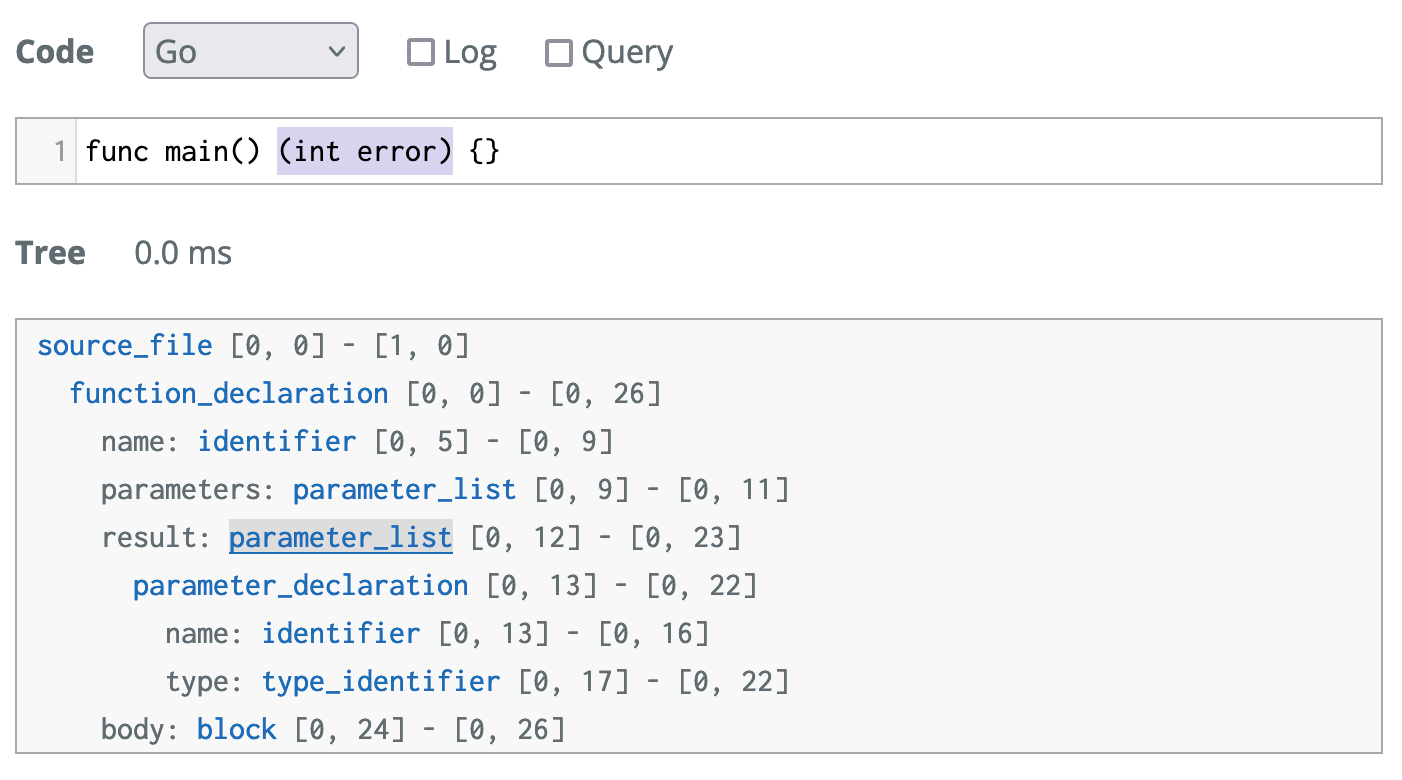# meain/blog

Oct 16, 2021 . 3 min

# Intelligent snippets using tree-sitter

I found myself writing a lot more go these days. So far I like the language even thought it seems way too simple at times. That said, one thing that I found a bit annoying was how I had to write all those default values when we have to return an error. I really liked how Rust handed errors, but yeah.

In this article I wanted to introduce a snippet that I use which will automatically fill in that `if err != nill` thing in your go program with all the proper return values for you. I actually got this idea from TJ DeVries, a Neovim core contributor.

Let me first show you the code and then we can go over it.

``(defun meain/go-default-returns (type)    "Making it a function instead of an alist so that we can handle unknown TYPE."    (pcase type        ("error" "err")        ("string" "\"\"")        ("rune" "0")        ("int" "0")        ("float64" "0.0")        ("bool" "false")        ("chan" "nil")        ((pred (string-prefix-p "<-")) "nil") ; channels        ((pred (string-prefix-p "[")) "nil") ; arrays        ((pred (string-match " ")) nil) ; for situations with return name        (_ (concat type "{}"))))(defun meain/go-return-string ()  "Get return string for go by looking up the return type of current func."  (let* ((func-node (tree-sitter-node-at-point 'function_declaration))         (return-node (tsc-get-child-by-field func-node ':result)))    ;; remove extra whitespace if nothing at end    (replace-regexp-in-string " \$" ""      (concat "return "        (if return-node          (let ((return-node-type (tsc-node-type return-node))                (return-node-text (tsc-node-text return-node)))            (pcase return-node-type                ('parameter_list (string-join                                    (remove-if (lambda (x) (equal nil x))                                     (mapcar 'meain/go-default-returns                                       (mapcar 'string-trim                                         (split-string (string-trim return-node-text "(" ")") ","))))                                   ", "))                (_ (meain/go-default-returns return-node-text)))))))))``

You can find it in my dotfiles

Here is what the code does, the first function `meain/go-default-returns` is a lookup table mapping each type to its default return value. The second function `meain/go-return-string` is what does the bulk of the work.

``(defun meain/go-return-string ()  "Get return string for go by looking up the return type of current func."  (let* ((func-node (tree-sitter-node-at-point 'function_declaration))         (return-node (tsc-get-child-by-field func-node ':result)))    ;; remove extra whitespace if nothing at end    (replace-regexp-in-string " \$" ""      (concat "return "        (if return-node          (let ((return-node-type (tsc-node-type return-node))                (return-node-text (tsc-node-text return-node)))            (pcase return-node-type                ('parameter_list (string-join                                    (remove-if (lambda (x) (equal nil x))                                     (mapcar 'meain/go-default-returns                                       (mapcar 'string-trim                                         (split-string (string-trim return-node-text "(" ")") ","))))                                   ", "))                (_ (meain/go-default-returns return-node-text)))))))))``

The 2nd and 3rd lines (the ones highlighted above) does the initial tree-sitter query. It first find out the function that we are in, and then looks up the result section by its label. You can check the tree sitter tree using the online playground. Here is what a sample go code will look like.``(defun meain/go-return-string ()  "Get return string for go by looking up the return type of current func."  (let* ((func-node (tree-sitter-node-at-point 'function_declaration))         (return-node (tsc-get-child-by-field func-node ':result)))    ;; remove extra whitespace if nothing at end    (replace-regexp-in-string " \$" ""      (concat "return "        (if return-node          (let ((return-node-type (tsc-node-type return-node))                (return-node-text (tsc-node-text return-node)))            (pcase return-node-type                ('parameter_list (string-join                                    (remove-if (lambda (x) (equal nil x))                                     (mapcar 'meain/go-default-returns                                       (mapcar 'string-trim                                         (split-string                                           (string-trim return-node-text "(" ")") ","))))                                   ", "))                (_ (meain/go-default-returns return-node-text)))))))))``

Now, if we are actually able to find a node, we continue and query tree-sitter to find out what kind of node it is and the actual content. We need to get the node type so that we can determine if it is just a single return or if there are multiple items to return.

``(defun meain/go-return-string ()  "Get return string for go by looking up the return type of current func."  (let* ((func-node (tree-sitter-node-at-point 'function_declaration))         (return-node (tsc-get-child-by-field func-node ':result)))    ;; remove extra whitespace if nothing at end    (replace-regexp-in-string " \$" ""      (concat "return "        (if return-node          (let ((return-node-type (tsc-node-type return-node))                (return-node-text (tsc-node-text return-node)))            (pcase return-node-type                ('parameter_list (string-join                                    (remove-if (lambda (x) (equal nil x))                                     (mapcar 'meain/go-default-returns                                       (mapcar 'string-trim                                         (split-string                                           (string-trim return-node-text "(" ")") ","))))                                   ", "))                (_ (meain/go-default-returns return-node-text)))))))))``

After that, we do a conditional and separately handle single vs multi entry ones. If there are multiple entries, the node-type will be `parameter_list` and we handle that in the first section. Let's get to that later. If it is not that, then we have the simple case of a single return statement for which we can use the above function we wrote to get the return, which is then prepended with "return " to get the final output.

In the case where we have multiple items, you have to read it inside out.

• strip "(" and ")"
• split string by ","
• trim any spaces
• map the function we wrote to get default over the values
• join them up with ", "

And we are good to go. Now that we have this, chuck in a `if err != nill {}` around it and wire up to your favorite snippet expansion library and you are good to go. I personally use auto-activating-snippets and you can find my setup here.

Hopefully you found that useful ;)

← Home